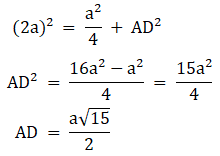# R S Aggarwal Solutions for Class 10 Maths Chapter 7 Triangles Exercise 7D

R S Aggarwal Solutions for class 10 Maths chapter 7 exercise 7D is available here. R S Aggarwal Solutions Class 10 Maths designed by experts at BYJU’s to help students. Subject experts have used simple and easy language to explain the concepts for better understanding. Students are advised to download R S Aggarwal Class 10 chapter 7 and practice more questions.

## Download PDF of R S Aggarwal Solutions for Class 10 Maths Chapter 7 Triangles Exercise 7D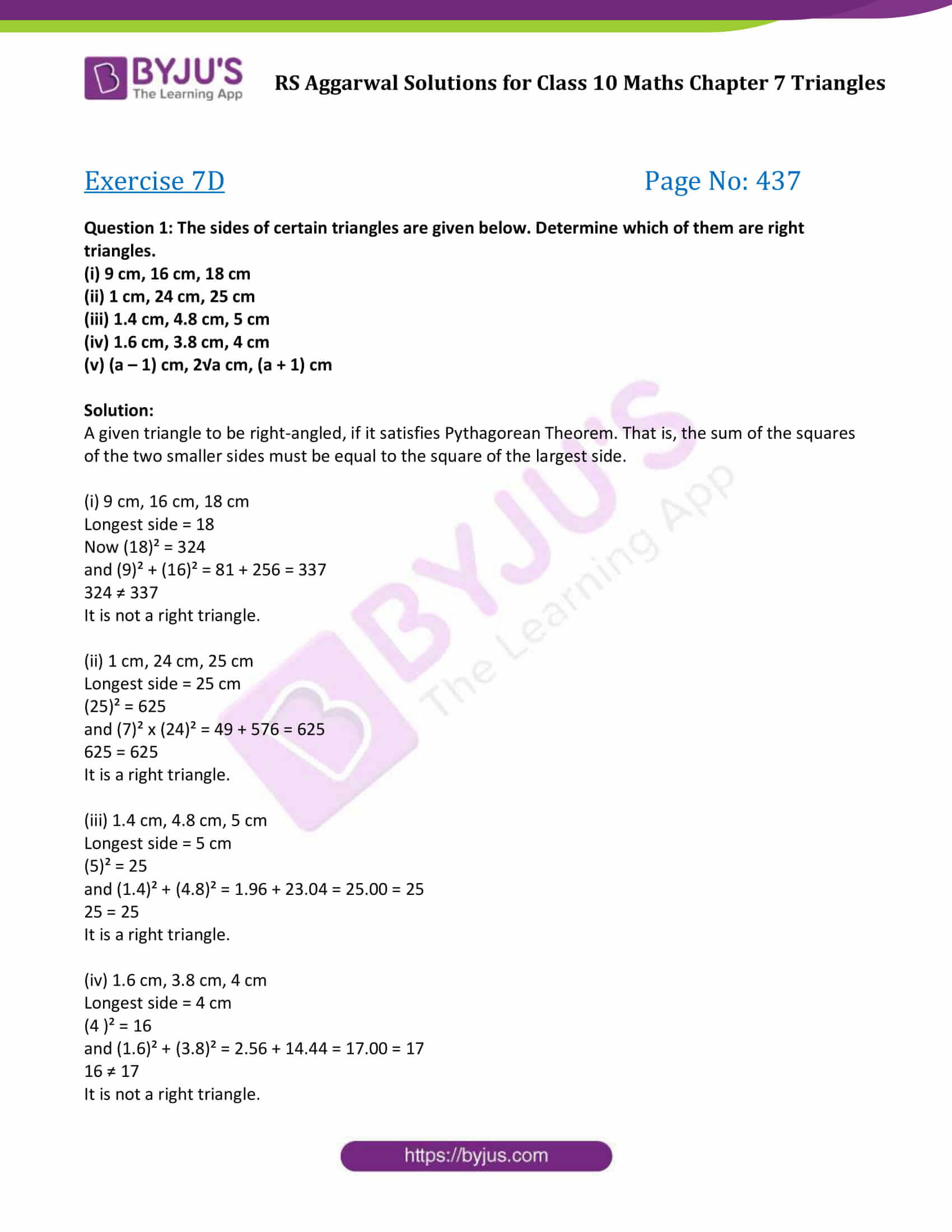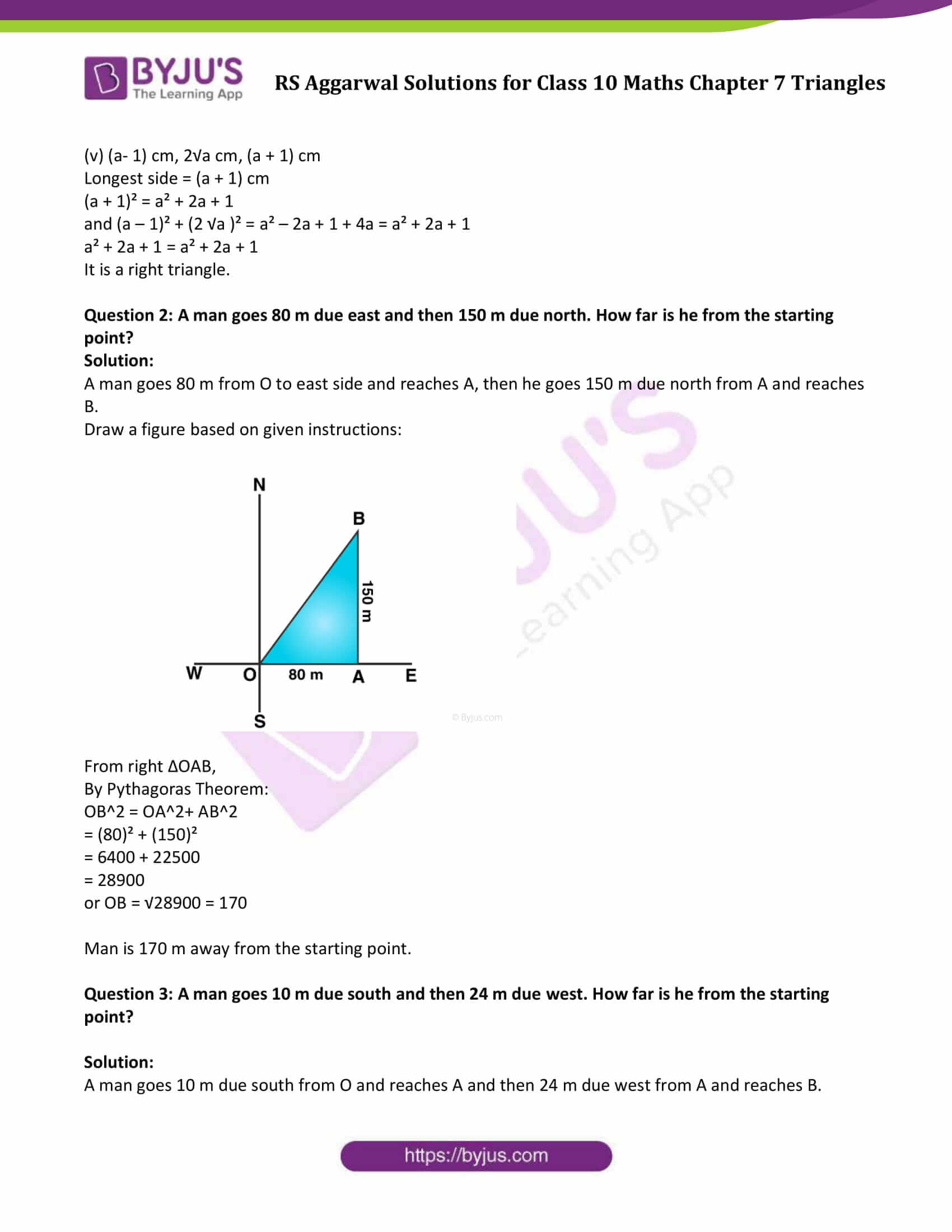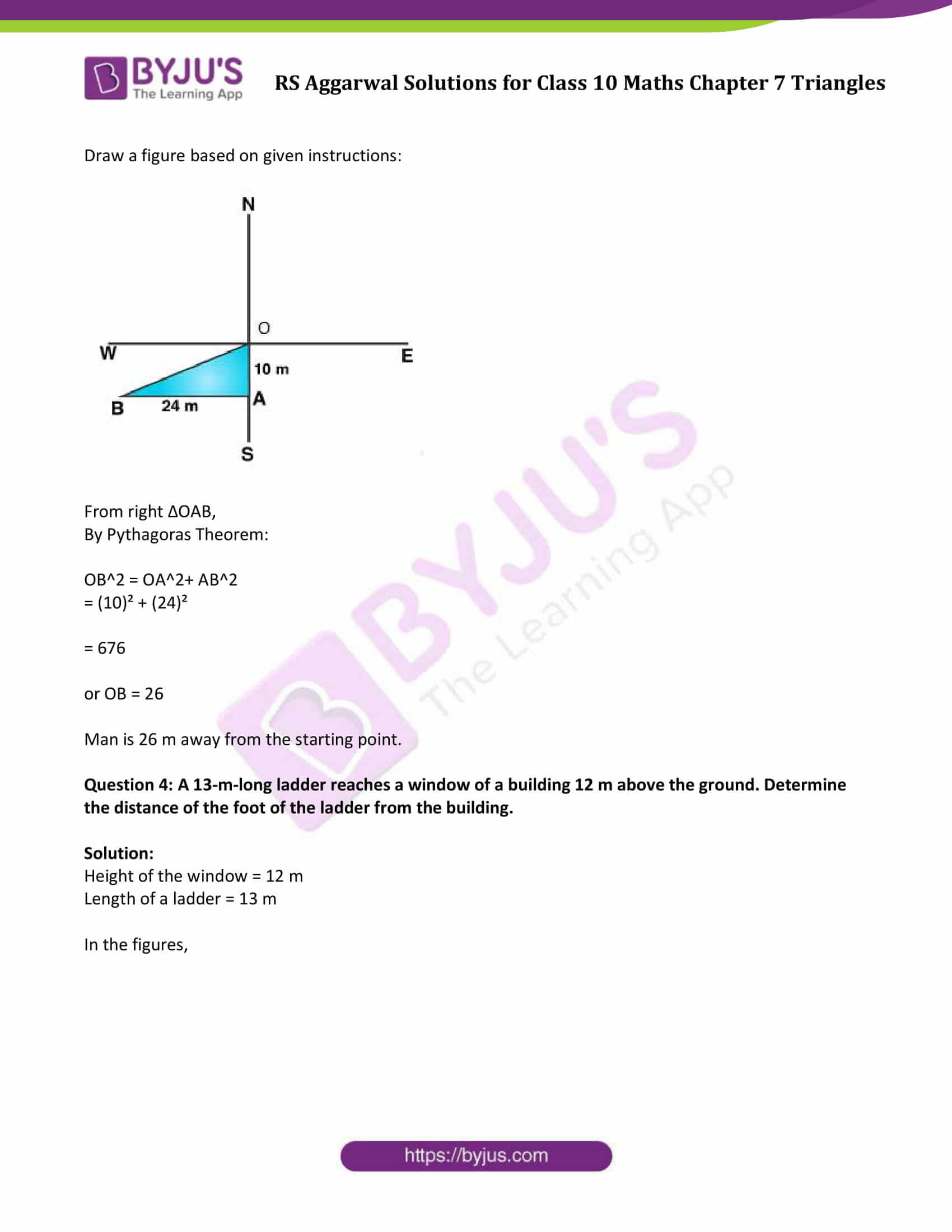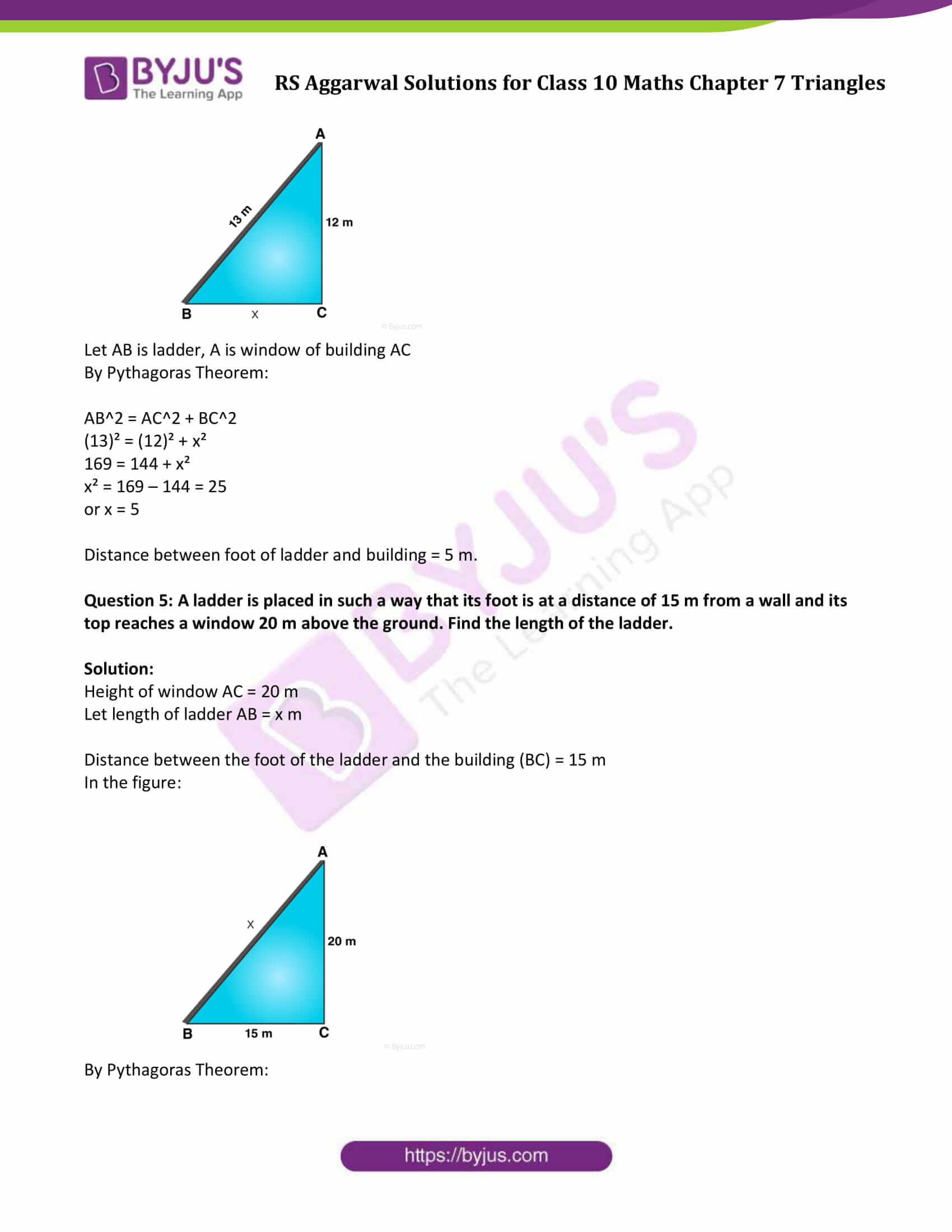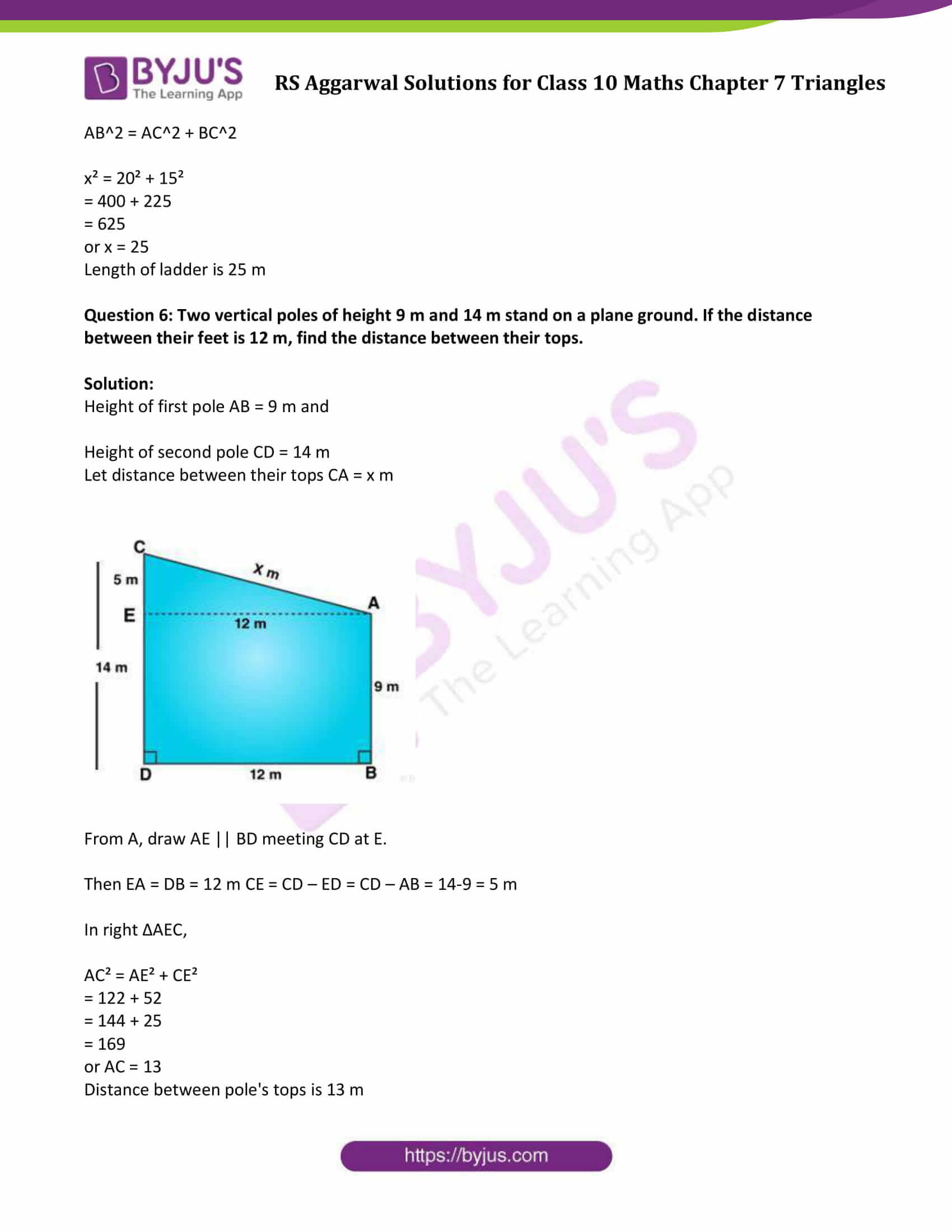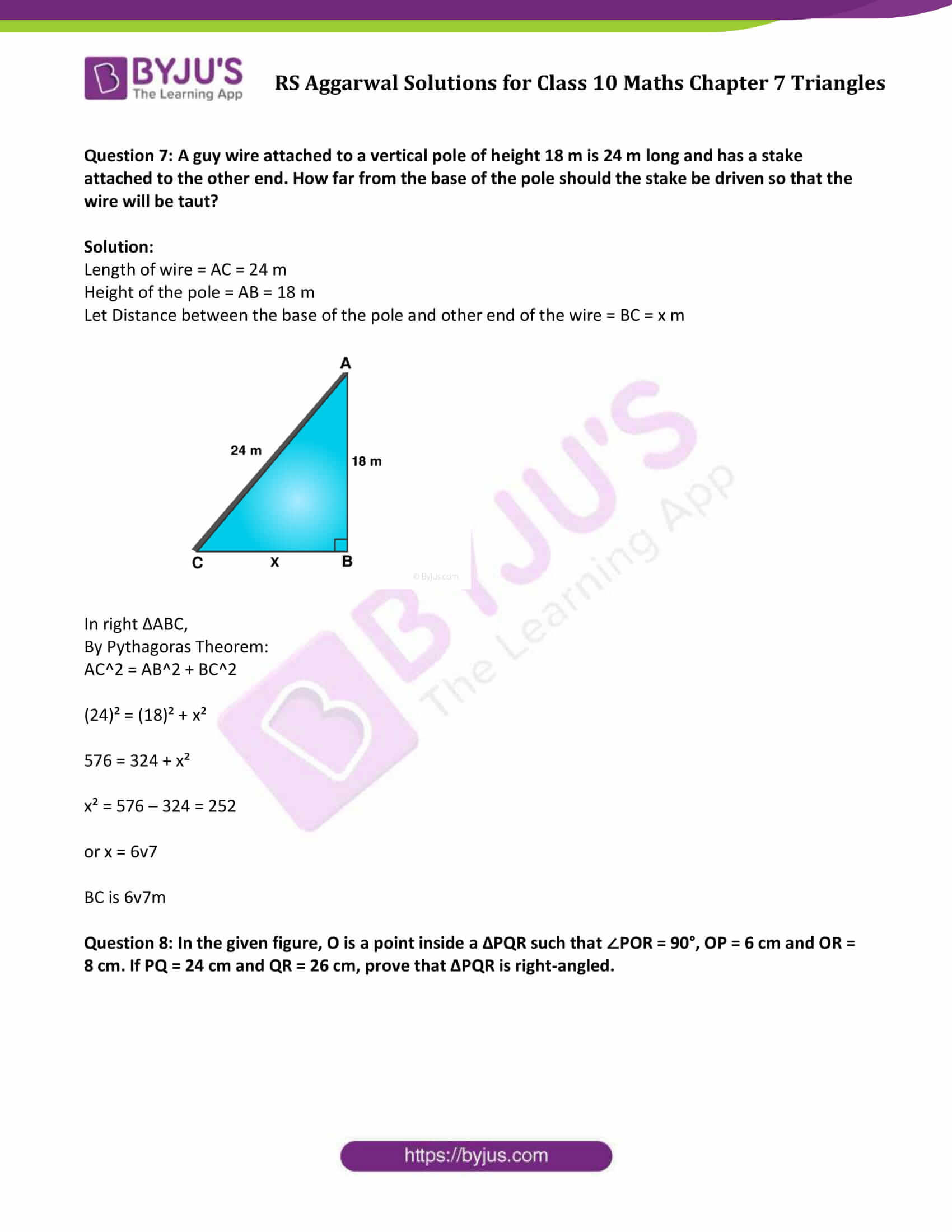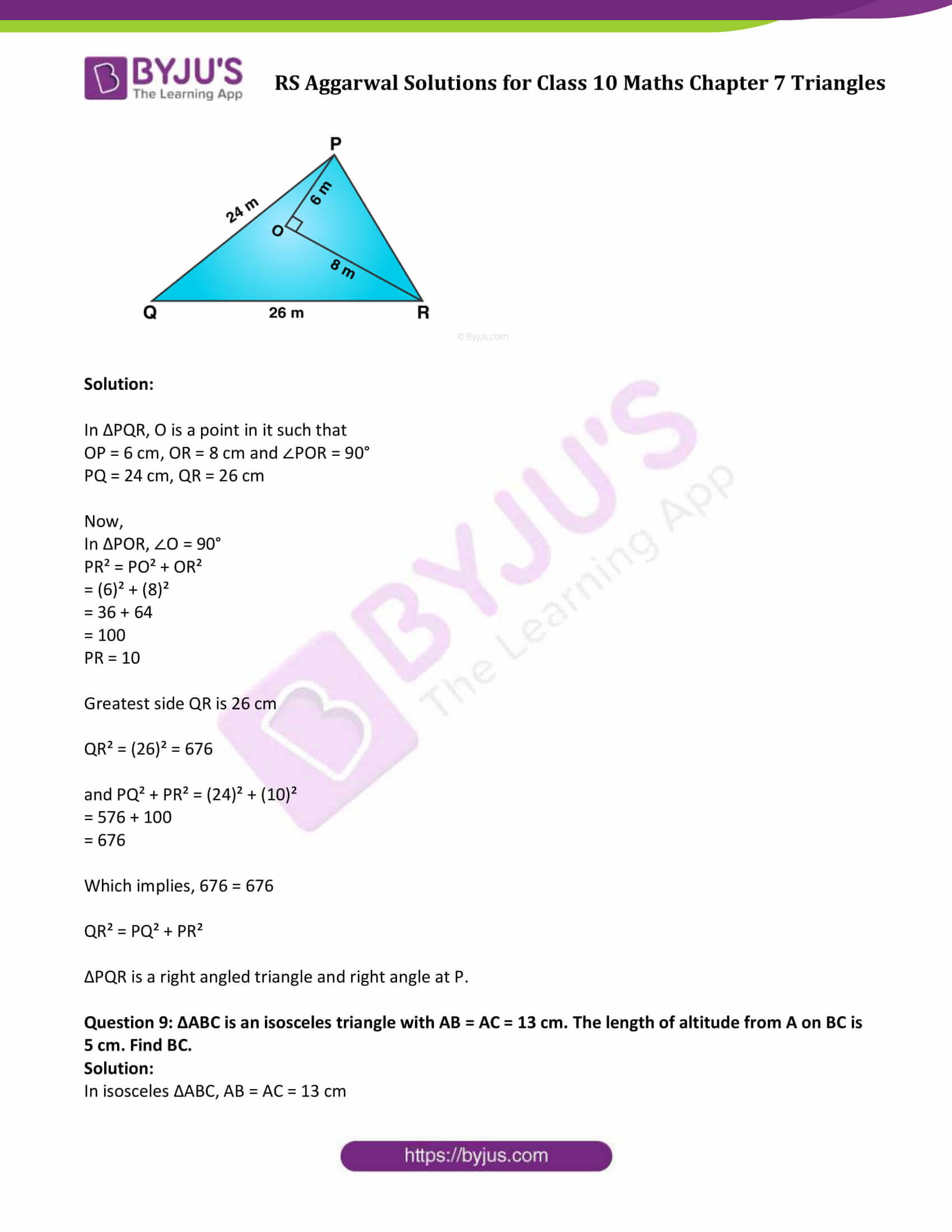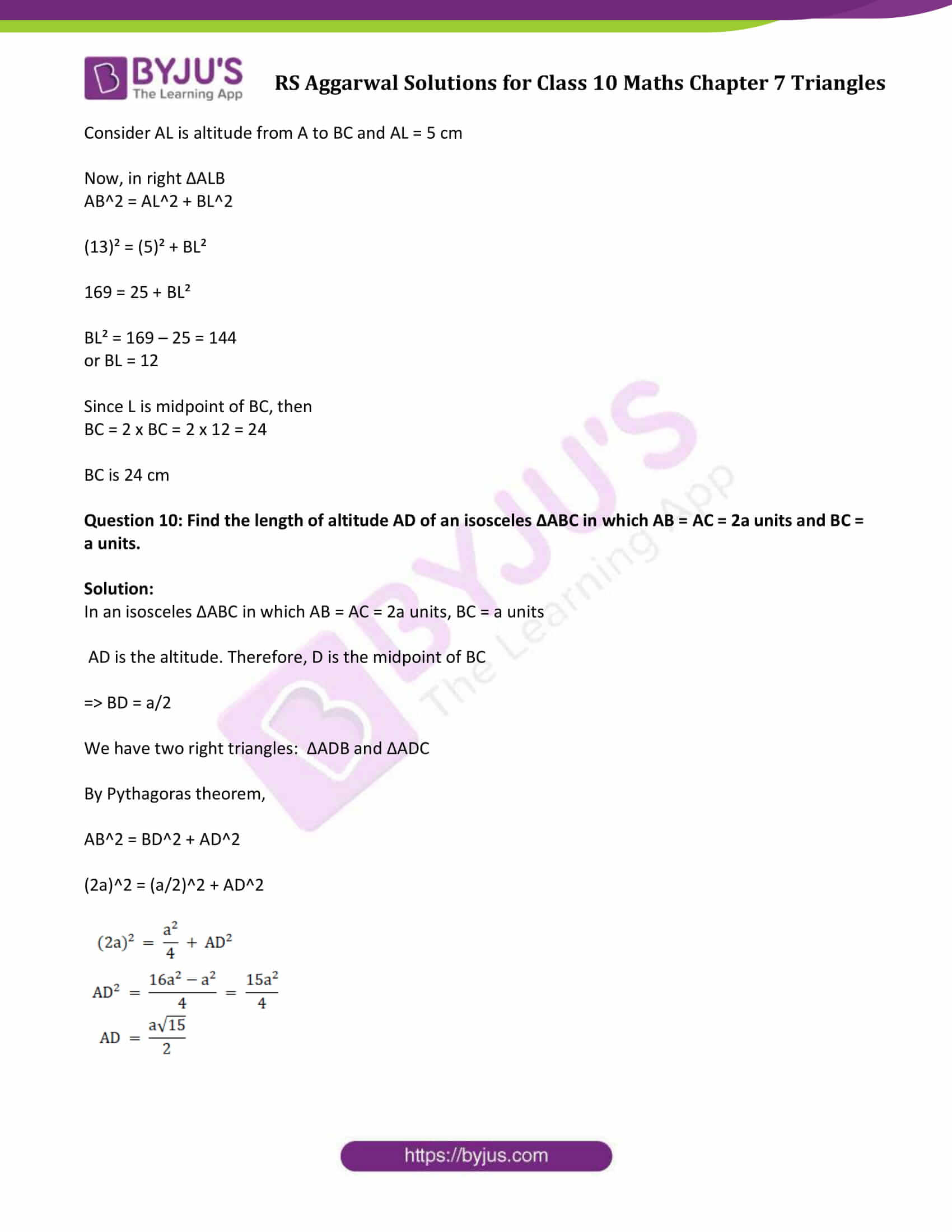### Access other exercise solutions of Class 10 Maths Chapter 7 Triangles

Exercise 7 A Solutions

Exercise 7 B Solutions

Exercise 7 C Solutions

Exercise 7 E Solutions

Question 1: The sides of certain triangles are given below. Determine which of them are right triangles.

(i) 9 cm, 16 cm, 18 cm

(ii) 1 cm, 24 cm, 25 cm

(iii) 1.4 cm, 4.8 cm, 5 cm

(iv) 1.6 cm, 3.8 cm, 4 cm

(v) (a – 1) cm, 2√a cm, (a + 1) cm

Solution:

A given triangle to be right-angled, if it satisfies Pythagorean Theorem. That is, the sum of the squares of the two smaller sides must be equal to the square of the largest side.

(i) 9 cm, 16 cm, 18 cm

Longest side = 18

Now (18)² = 324

and (9)² + (16)² = 81 + 256 = 337

324 ≠ 337

It is not a right triangle.

(ii) 1 cm, 24 cm, 25 cm

Longest side = 25 cm

(25)² = 625

and (7)² x (24)² = 49 + 576 = 625

625 = 625

It is a right triangle.

(iii) 1.4 cm, 4.8 cm, 5 cm

Longest side = 5 cm

(5)² = 25

and (1.4)² + (4.8)² = 1.96 + 23.04 = 25.00 = 25

25 = 25

It is a right triangle.

(iv) 1.6 cm, 3.8 cm, 4 cm

Longest side = 4 cm

(4 )² = 16

and (1.6)² + (3.8)² = 2.56 + 14.44 = 17.00 = 17

16 ≠ 17

It is not a right triangle.

(v) (a- 1) cm, 2√a cm, (a + 1) cm

Longest side = (a + 1) cm

(a + 1)² = a² + 2a + 1

and (a – 1)² + (2 √a )² = a² – 2a + 1 + 4a = a² + 2a + 1

a² + 2a + 1 = a² + 2a + 1

It is a right triangle.

Question 2: A man goes 80 m due east and then 150 m due north. How far is he from the starting point?

Solution:

A man goes 80 m from O to east side and reaches A, then he goes 150 m due north from A and reaches B.

Draw a figure based on given instructions: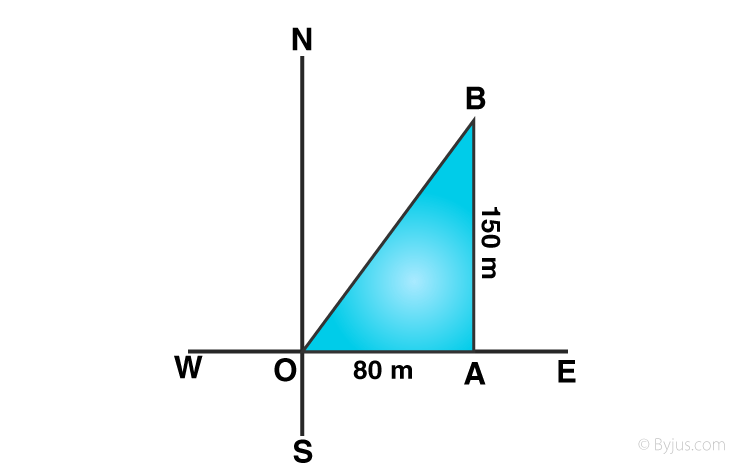From right ∆OAB,

By Pythagoras Theorem:

OB2 = OA2+ AB2

= (80)² + (150)²

= 6400 + 22500

= 28900

or OB = √28900 = 170

Man is 170 m away from the starting point.

Question 3: A man goes 10 m due south and then 24 m due west. How far is he from the starting point?

Solution:

A man goes 10 m due south from O and reaches A and then 24 m due west from A and reaches B.

Draw a figure based on given instructions: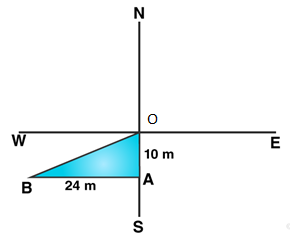From right ∆OAB,

By Pythagoras Theorem:

OB2 = OA2+ AB2

= (10)² + (24)²

= 676

or OB = 26

Man is 26 m away from the starting point.

Question 4: A 13-m-long ladder reaches a window of a building 12 m above the ground. Determine the distance of the foot of the ladder from the building.

Solution:

Height of the window = 12 m

Length of a ladder = 13 m

In the figures,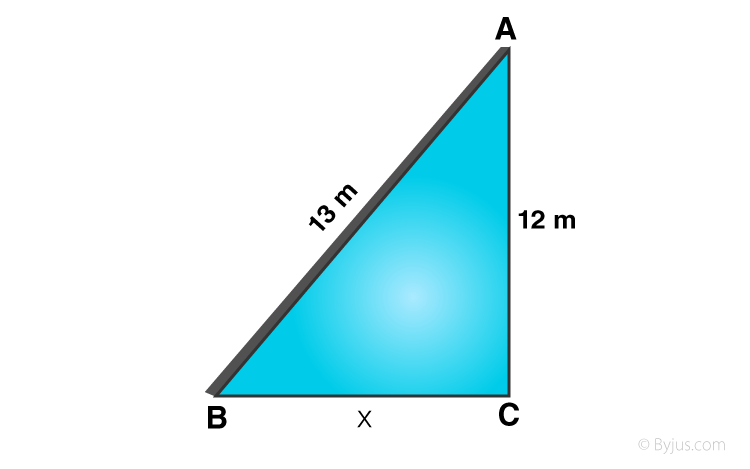Let AB is ladder, A is window of building AC

By Pythagoras Theorem:

AB2 = AC2 + BC2

(13)² = (12)² + x²

169 = 144 + x²

x² = 169 – 144 = 25

or x = 5

Distance between foot of ladder and building = 5 m.

Question 5: A ladder is placed in such a way that its foot is at a distance of 15 m from a wall and its top reaches a window 20 m above the ground. Find the length of the ladder.

Solution:

Height of window AC = 20 m

Let length of ladder AB = x m

Distance between the foot of the ladder and the building (BC) = 15 m

In the figure: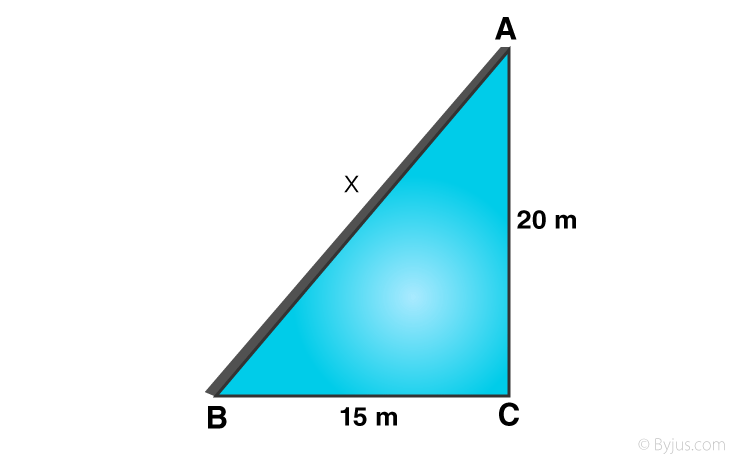By Pythagoras Theorem:

AB2 = AC2 + BC2

x² = 20² + 15²

= 400 + 225

= 625

or x = 25

Length of ladder is 25 m

Question 6: Two vertical poles of height 9 m and 14 m stand on a plane ground. If the distance between their feet is 12 m, find the distance between their tops.

Solution:

Height of first pole AB = 9 m and

Height of second pole CD = 14 m

Let distance between their tops CA = x m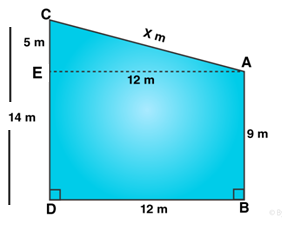From A, draw AE || BD meeting CD at E.

Then EA = DB = 12 m CE = CD – ED = CD – AB = 14-9 = 5 m

In right ∆AEC,

AC² = AE² + CE²

= 122 + 52

= 144 + 25

= 169

or AC = 13

Distance between pole’s tops is 13 m

Question 7: A guy wire attached to a vertical pole of height 18 m is 24 m long and has a stake attached to the other end. How far from the base of the pole should the stake be driven so that the wire will be taut?

Solution:

Length of wire = AC = 24 m

Height of the pole = AB = 18 m

Let Distance between the base of the pole and other end of the wire = BC = x m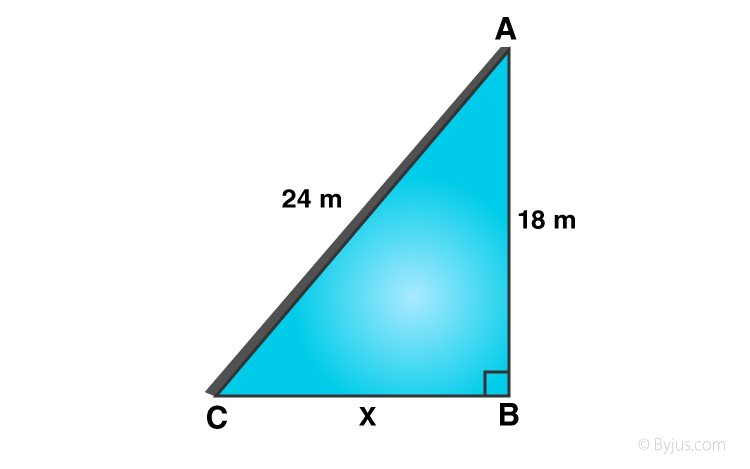In right ∆ABC,

By Pythagoras Theorem:

AC2 = AB2 + BC2

(24)² = (18)² + x²

576 = 324 + x²

x² = 576 – 324 = 252

or x = 6v7

BC is 6v7m

Question 8: In the given figure, O is a point inside a ∆PQR such that ∠POR = 90°, OP = 6 cm and OR = 8 cm. If PQ = 24 cm and QR = 26 cm, prove that ∆PQR is right-angled.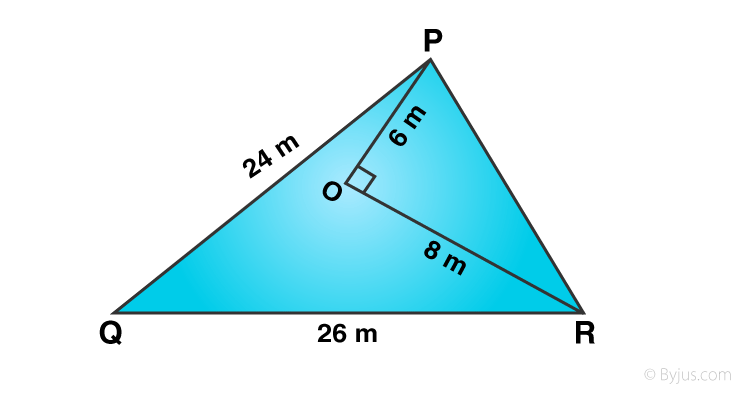Solution:

In ∆PQR, O is a point in it such that

OP = 6 cm, OR = 8 cm and ∠POR = 90°

PQ = 24 cm, QR = 26 cm

Now,

In ∆POR, ∠O = 90°

PR² = PO² + OR²

= (6)² + (8)²

= 36 + 64

= 100

PR = 10

Greatest side QR is 26 cm

QR² = (26)² = 676

and PQ² + PR² = (24)² + (10)²

= 576 + 100

= 676

Which implies, 676 = 676

QR² = PQ² + PR²

∆PQR is a right angled triangle and right angle at P.

Question 9: ∆ABC is an isosceles triangle with AB = AC = 13 cm. The length of altitude from A on BC is 5 cm. Find BC.

Solution:

In isosceles ∆ABC, AB = AC = 13 cm

Consider AL is altitude from A to BC and AL = 5 cm

Now, in right ∆ALB

AB2 = AL2 + BL2

(13)² = (5)² + BL²

169 = 25 + BL²

BL² = 169 – 25 = 144

or BL = 12

Since L is midpoint of BC, then

BC = 2 x BC = 2 x 12 = 24

BC is 24 cm

Question 10: Find the length of altitude AD of an isosceles ∆ABC in which AB = AC = 2a units and BC = a units.

Solution:

In an isosceles ∆ABC in which AB = AC = 2a units, BC = a units

AD is the altitude. Therefore, D is the midpoint of BC

=> BD = a/2

By Pythagoras theorem,